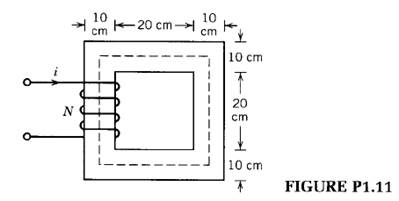### Create an Account

Already have account?

### Forgot Your Password ?

Home / Questions / Repeat Problem 111 for a 10 cm wide air gap in the core Assume a 10% increase in the effe...

# Repeat Problem 111 for a 10 cm wide air gap in the core Assume a 10% increase in the effective cross sectional area of the air gap due to fringing in the air gap Problem 111 The magnetic

Repeat Problem 1.11 for a 1.0 cm wide air gap in the core. Assume a 10% increase in the effective cross sectional area of the air gap due to fringing in the air gap.

Problem 1.11

The magnetic circuit of Fig. P1.11 has a core of relative permeability uT = 2000. The depth of the core is 5 cm. The coil has 400 turns and carries a current of 1.5 A.

(a)    Draw the magnetic equivalent circuit.

(b)    Find the flux and the flux density in the core.

(c)     Determine the inductance of the coil.Jul 16 2020 View more View Less

#### Answer (Solved)Subscribe To Get Solution